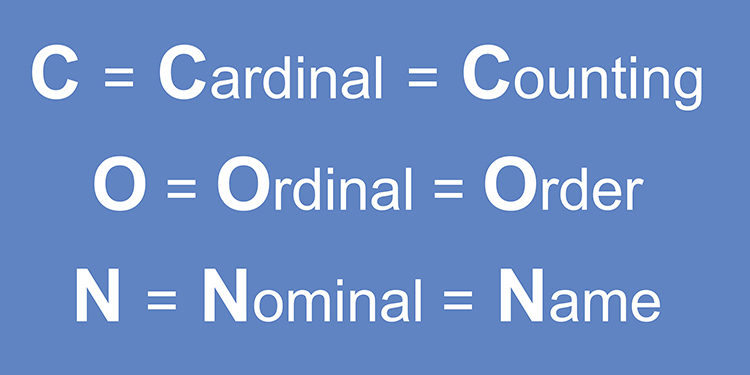# What’s the difference between ordinal and cardinal numbers?Cardinal numbers are the numbers that are used for counting. It helps us to know how many elements are there. Ordinal numbers are the concept of natural numbers which is used to describe a way to arrange different elements
• Ordinal numbers are those used to indicate order or position, such as "first," "second," "third," etc.
• Cardinal numbers are those used to count, such as "one," "two," "three," etc.

So when we say that someone is the "third person in line," we are using an ordinal number.

And when we say that there are "three people in line," we are using a cardinal number.

• It's important to remember that ordinal numbers are always used with "the," whereas cardinal numbers are not. For example, you would say "the first person in line," but you would not say "the one person in line."

Tips

Here's a helpful tip: to remember the difference between ordinal and cardinal numbers, think of the word "cardinal" as related to the word "card" (as in a playing card). So if you can remember that ordinal numbers are related to the word "order," and cardinal numbers are related to the word "card," you'll never get the two confused again!